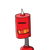# solve x+x/2+x/3<=5. represent the solution set on number line​

solve x+x/2+x/3<=5. represent the solution set on number line​

### 1 thought on “solve x+x/2+x/3<=5. represent the solution set on number line​”

1.Step-by-step explanation:

x+x/2+x/3≤5

3x/2+x/3≤5

9x/6+2x/6≤5

11x/6≤5

11x≤5×6=30

x≤30/11

Therefore on a number line the set would be smaller than equal to 30/11